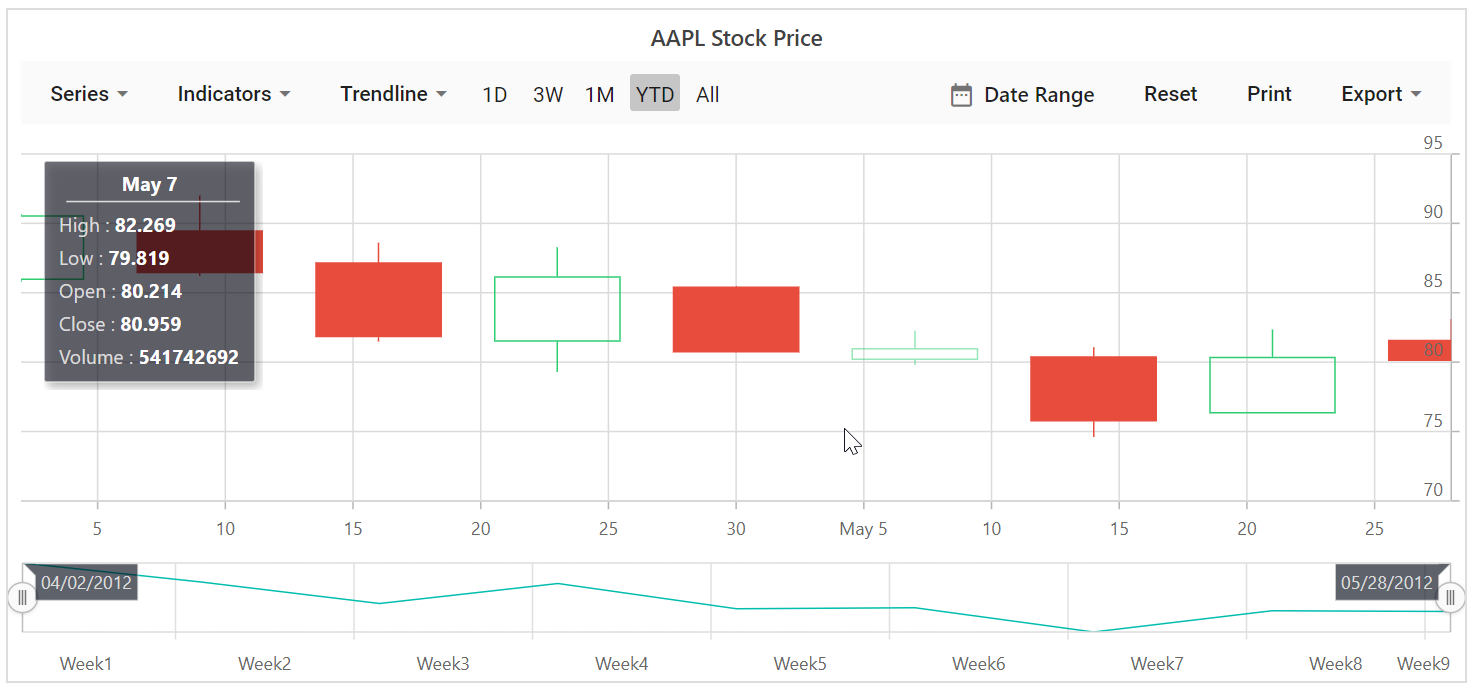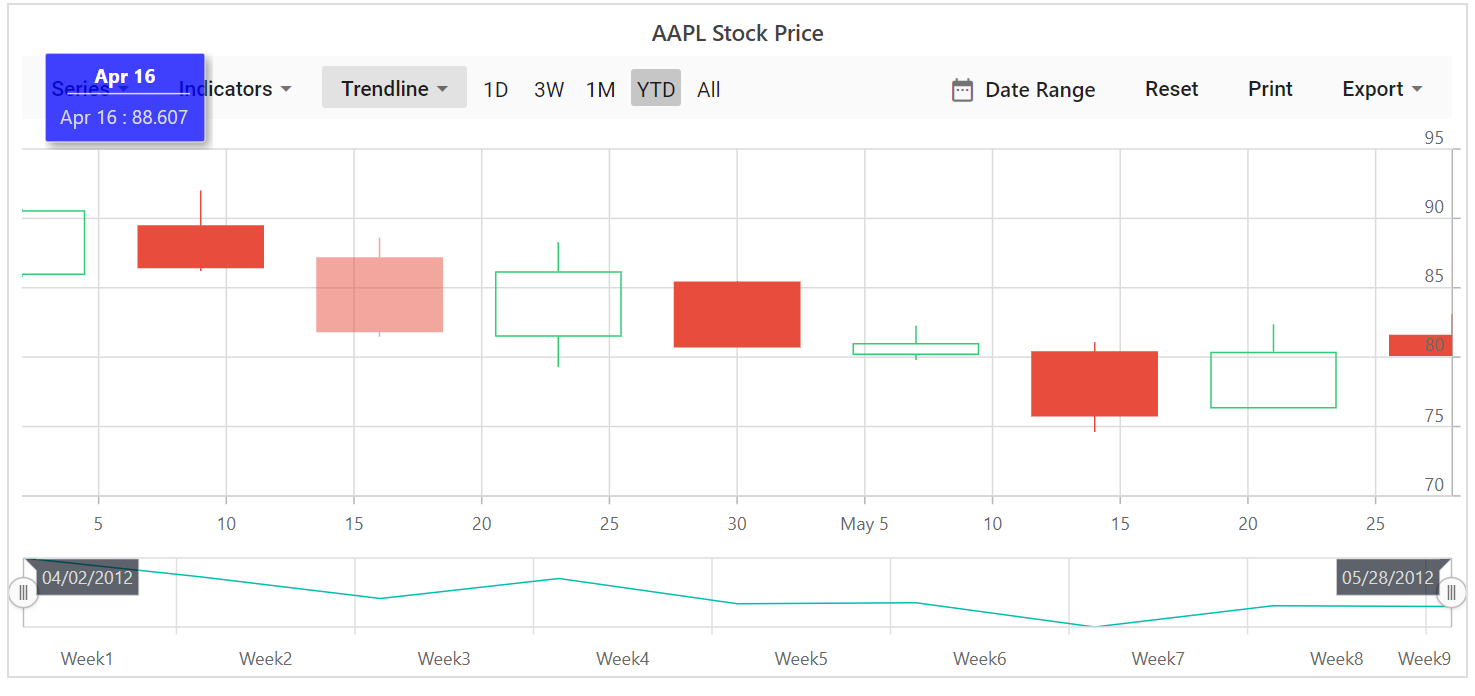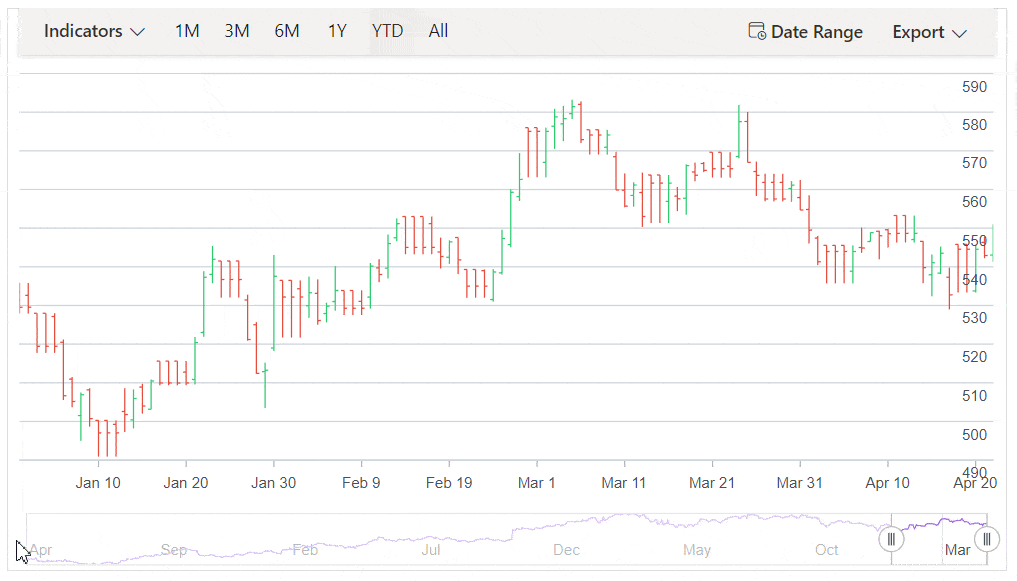# Tooltip in Blazor Stock Chart Component

25 Sep 202310 minutes to read

Stock Chart will display details about the points through tooltip, when the mouse is moved over the point.

## Default tooltip

By default, tooltip is not visible. Enable the tooltip by setting Enable property to true .

``````@using Syncfusion.Blazor.Charts

<SfStockChart Title="AAPL Stock Price">
<StockChartTooltipSettings Enable="true">
</StockChartTooltipSettings>

<StockChartSeriesCollection>
<StockChartSeries DataSource="@StockDetails" Type="ChartSeriesType.Candle" XName="Date" High="High" Low="Low" Open="Open" Close="Close" Volume="Volume" Name="Google"></StockChartSeries>
</StockChartSeriesCollection>
</SfStockChart>

@code {

public class ChartData
{
public DateTime Date { get; set; }
public Double Open { get; set; }
public Double Low { get; set; }
public Double Close { get; set; }
public Double High { get; set; }
public Double Volume { get; set; }
}

public List<ChartData> StockDetails = new List<ChartData>
{
new ChartData { Date = new DateTime(2012, 04, 02), Open= 85.9757, High = 90.6657,Low = 85.7685, Close = 90.5257,Volume = 660187068},
new ChartData { Date = new DateTime(2012, 04, 09), Open= 89.4471, High = 92,Low = 86.2157, Close = 86.4614,Volume = 912634864},
new ChartData { Date = new DateTime(2012, 04, 16), Open= 87.1514, High = 88.6071,Low = 81.4885, Close = 81.8543,Volume = 1221746066},
new ChartData { Date = new DateTime(2012, 04, 23), Open= 81.5157, High = 88.2857,Low = 79.2857, Close = 86.1428,Volume = 965935749},
new ChartData { Date = new DateTime(2012, 04, 30), Open= 85.4, High =  85.4857,Low = 80.7385, Close = 80.75,Volume = 615249365},
new ChartData { Date = new DateTime(2012, 05, 07), Open= 80.2143, High = 82.2685,Low = 79.8185, Close = 80.9585,Volume = 541742692},
new ChartData { Date = new DateTime(2012, 05, 14), Open= 80.3671, High = 81.0728,Low = 74.5971, Close = 75.7685,Volume = 708126233},
new ChartData { Date = new DateTime(2012, 05, 21), Open= 76.3571, High = 82.3571,Low = 76.2928, Close = 80.3271,Volume = 682076215},
new ChartData { Date = new DateTime(2012, 05, 28), Open= 81.5571, High = 83.0714,Low = 80.0743, Close = 80.1414,Volume = 480059584}
};
}``````## Format the tooltip

By default, tooltip shows information of x and y value in points. In addition to that, more information can be shown in tooltip. For example the format `\${point.x} : \${point.high}` shows point x and high value.

``````@using Syncfusion.Blazor.Charts

<SfStockChart Title="AAPL Stock Price">
<StockChartTooltipSettings Enable="true" Format="<b>\${point.x} : \${point.high}</b>">
</StockChartTooltipSettings>

<StockChartSeriesCollection>
<StockChartSeries DataSource="@StockDetails" Type="ChartSeriesType.Candle" XName="Date" High="High" Low="Low" Open="Open" Close="Close" Volume="Volume" Name="google"></StockChartSeries>
</StockChartSeriesCollection>
</SfStockChart>

@code {

public class ChartData
{
public DateTime Date { get; set; }
public Double Open { get; set; }
public Double Low { get; set; }
public Double Close { get; set; }
public Double High { get; set; }
public Double Volume { get; set; }
}

public List<ChartData> StockDetails = new List<ChartData>
{
new ChartData { Date = new DateTime(2012, 04, 02), Open= 85.9757, High = 90.6657,Low = 85.7685, Close = 90.5257,Volume = 660187068},
new ChartData { Date = new DateTime(2012, 04, 09), Open= 89.4471, High = 92,Low = 86.2157, Close = 86.4614,Volume = 912634864},
new ChartData { Date = new DateTime(2012, 04, 16), Open= 87.1514, High = 88.6071,Low = 81.4885, Close = 81.8543,Volume = 1221746066},
new ChartData { Date = new DateTime(2012, 04, 23), Open= 81.5157, High = 88.2857,Low = 79.2857, Close = 86.1428,Volume = 965935749},
new ChartData { Date = new DateTime(2012, 04, 30), Open= 85.4, High =  85.4857,Low = 80.7385, Close = 80.75,Volume = 615249365},
new ChartData { Date = new DateTime(2012, 05, 07), Open= 80.2143, High = 82.2685,Low = 79.8185, Close = 80.9585,Volume = 541742692},
new ChartData { Date = new DateTime(2012, 05, 14), Open= 80.3671, High = 81.0728,Low = 74.5971, Close = 75.7685,Volume = 708126233},
new ChartData { Date = new DateTime(2012, 05, 21), Open= 76.3571, High = 82.3571,Low = 76.2928, Close = 80.3271,Volume = 682076215},
new ChartData { Date = new DateTime(2012, 05, 28), Open= 81.5571, High = 83.0714,Low = 80.0743, Close = 80.1414,Volume = 480059584}
};
}``````

## Customize the appearance of tooltip

The Fill and Border properties are used to customize the background color and border of the tooltip respectively. The TextStyle property in the tooltip is used to customize the font of the tooltip text.

``````@using Syncfusion.Blazor.Charts

<SfStockChart Title="AAPL Stock Price">
<StockChartTooltipSettings Enable="true" Format="\${point.x} : \${point.high}" Fill="#7bb4eb">
</StockChartTooltipSettings>

<StockChartSeriesCollection>
<StockChartSeries DataSource="@StockDetails" Type="ChartSeriesType.Candle" XName="Date" High="High" Low="Low" Open="Open" Close="Close" Volume="Volume" Name="Google"></StockChartSeries>
</StockChartSeriesCollection>
</SfStockChart>

@code {

public class ChartData
{
public DateTime Date { get; set; }
public Double Open { get; set; }
public Double Low { get; set; }
public Double Close { get; set; }
public Double High { get; set; }
public Double Volume { get; set; }
}

public List<ChartData> StockDetails = new List<ChartData>
{
new ChartData { Date = new DateTime(2012, 04, 02), Open= 85.9757, High = 90.6657,Low = 85.7685, Close = 90.5257,Volume = 660187068},
new ChartData { Date = new DateTime(2012, 04, 09), Open= 89.4471, High = 92,Low = 86.2157, Close = 86.4614,Volume = 912634864},
new ChartData { Date = new DateTime(2012, 04, 16), Open= 87.1514, High = 88.6071,Low = 81.4885, Close = 81.8543,Volume = 1221746066},
new ChartData { Date = new DateTime(2012, 04, 23), Open= 81.5157, High = 88.2857,Low = 79.2857, Close = 86.1428,Volume = 965935749},
new ChartData { Date = new DateTime(2012, 04, 30), Open= 85.4, High =  85.4857,Low = 80.7385, Close = 80.75,Volume = 615249365},
new ChartData { Date = new DateTime(2012, 05, 07), Open= 80.2143, High = 82.2685,Low = 79.8185, Close = 80.9585,Volume = 541742692},
new ChartData { Date = new DateTime(2012, 05, 14), Open= 80.3671, High = 81.0728,Low = 74.5971, Close = 75.7685,Volume = 708126233},
new ChartData { Date = new DateTime(2012, 05, 21), Open= 76.3571, High = 82.3571,Low = 76.2928, Close = 80.3271,Volume = 682076215},
new ChartData { Date = new DateTime(2012, 05, 28), Open= 81.5571, High = 83.0714,Low = 80.0743, Close = 80.1414,Volume = 480059584}
};
}``````## Tooltip position

By default, the tooltip appears on the left side of the stock chart. When moving the mouse pointer across data points, you can move the tooltip along with the mouse by setting `Nearest` to the `TooltipPosition` property in the StockChartTooltipSetting.

``````@using Syncfusion.Blazor
@using Syncfusion.Blazor.Charts
@using System.Net.Http.Json
@inject HttpClient Http

@if (dataSource == null)
{
</div>
}
else
{
<SfStockChart>
<StockChartPrimaryXAxis>
<StockChartAxisMajorGridLines Width="0"></StockChartAxisMajorGridLines>
<StockChartAxisCrosshairTooltip Enable="true"></StockChartAxisCrosshairTooltip>
</StockChartPrimaryXAxis>
<StockChartPrimaryYAxis LabelFormat="n0">
<StockChartAxisLineStyle Width="0"></StockChartAxisLineStyle>
<StockChartAxisMajorTickLines Width="0"></StockChartAxisMajorTickLines>
</StockChartPrimaryYAxis>
<StockChartTooltipSettings Enable="true" TooltipPosition="TooltipPosition.Nearest"></StockChartTooltipSettings>
<StockChartCrosshairSettings Enable="true"></StockChartCrosshairSettings>
<StockChartSeriesCollection>
<StockChartSeries DataSource="@dataSource" Type="ChartSeriesType.HiloOpenClose" XName="x"></StockChartSeries>
</StockChartSeriesCollection>
<StockChartChartArea>
<StockChartChartAreaBorder Width="0"></StockChartChartAreaBorder>
</StockChartChartArea>
</SfStockChart>
}
@code{
private ChartData[] dataSource;

{
dataSource = await Http.GetFromJsonAsync<ChartData[]>(NavigationManager.BaseUri + "./googl.json");
}

public class ChartData
{
public DateTime x { get; set; }
public double open { get; set; }
public double low { get; set; }
public double close { get; set; }
public double high { get; set; }
public double volume { get; set; }
}
}``````NOTE

Refer to our Blazor Stock Charts feature tour page for its groundbreaking feature representations and also explore our Blazor Stock Chart Example to know various stock chart types and how to represent time-dependent data, showing trends at equal intervals.